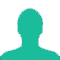600 students took the test on Physics and chemistry. 35% students failed in Physics and 45% students failed in chemistry and 40% of those who passed in chemistry also passed in Physics, then how many students failed in both :

A. 16

B. 15

C. 13

D. 12

Solution(By Examveda Team)

 Result Physics Chemistry Failed 35% 45% Passed 65% 55%

Now, 55% of 600 passed in Chemistry = $$\frac{{55 \times 600}}{{100}}$$   = 330
From question, 40% of those who passed in Chemistry(330 Students) also passed in physics
= $$\frac{{40 \times 330}}{{100}}$$
= 132,
so, 132 students passed in both
Therefore % who passed in both = $$\frac{{132 \times 100}}{{600}} = 22\%$$
Passed in both subject = 22% of total students
% of students who passed in either Physics or Chemistry or both,
= (65 + 55) - 22
= 98%
Thus, percentage of students who failed in both subjects = 2%
Number of students who failed = 2% of 600 = 12

1.Physics failed = 35%,Pass = 65%
Chemistry failed = 45%, Pass = 55%, Both Pass = 40% of 55% = 22%
Total failed = 78%
so,
78% = 35%+ 45% - both
both = 2%

600 of 2%
= 12

2.How 22% came?

3.45% failed in chem => 55% passed in chem
35% failed in pay => 65% passed in pay

now, 55% of 600 passed in chem => 55/100*600 = 330
from question, 40% of those who passed in chem(i.e 330) also passed in pay => 40/100 *330 = 132 , so, 132 students passed in both
so % who passed in both = 132/600*100 = 22%
so, % of student who passed in any of the physics or chem or both (65% +55% -22%) =98%
so, the % of students who are failed in both =2% => 2/100*600 =>12

4.it is given that 40% of the students passed in both the subject how are u taking 22%

5.it is given that 40% of the students passed in both the subject how are u taking 22%

Related Questions on Percentage